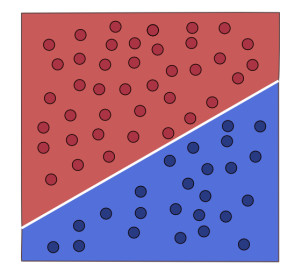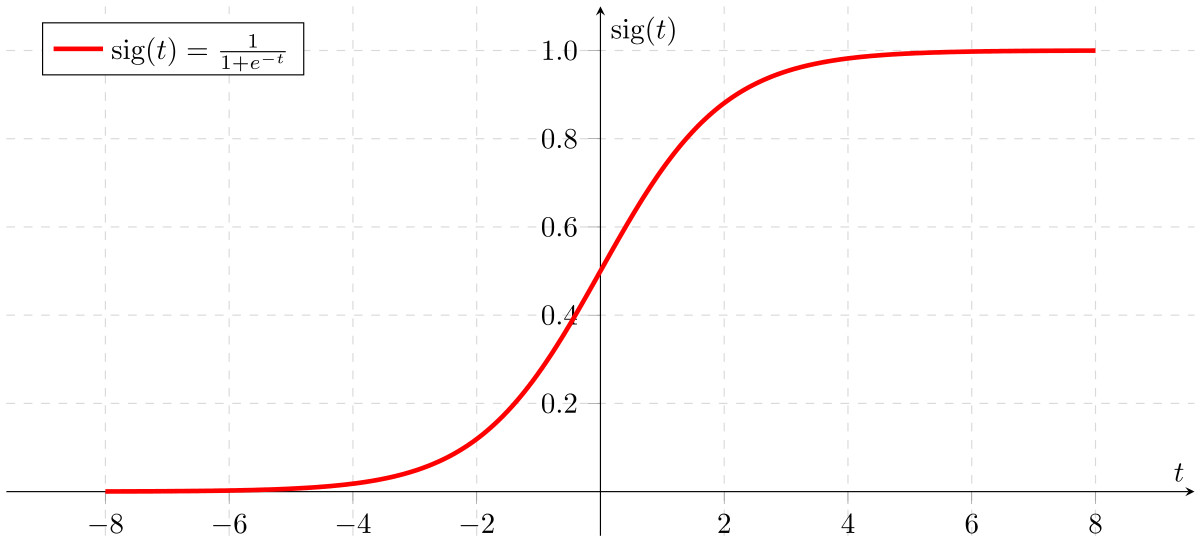April 23, 2019

# Logistic regression from scratch in Python

Logistic regression is a supervised learning algorithm used to resolve classification problems.

This algorithm is quite important if you want to learn about neural networks, we could say that this is the first step you should take in order to learn about neural networks.

I recommend you to read my post about decision trees since in that post I explain some important topics that we will see in this post.

## Dataset

We will use the dataset of people with diabetes again. This dataset contains information about 768 people, therefore we have 768 rows and 9 columns:

• Pregnancies

• Glucose

• BloodPressure

• SkinThickness

• Insulin

• BMI

• DiabetesPedigreeFunction

• Age

• Outcome

We use the outcome column to create the variable y, the remaining columns, that we call features, are used to create the variable X.

## Logistic regression model

We will see each part of the logistic regression model

### Probability function

``````
Z = WX + b

``````

This function uses the variables W and b to create a relationship between the data X and the model.

#### W

This variable called weights is used to denote how much each feature of the dataset X matters in the model, for example if the contribution of the feature Insulin is important to predict if the patient has diabetes or not, the model will assign a bigger value for this feature. As we saw, we use the features columns to create the variable X, for instance the variable W must have one value for each feature:

``````
W = [[0.3, 0.1, 0.6, 0.8, 0.4, 0.6, 0.7, 0.4]]

``````

#### b

This variable is called bias, if we print the logistic regression model we will have a line that splits the data into two classes:The parameter b moves this line from the origin point (0, 0), in the image above b has a value of 3, if b had a value of 0 the line would begin at the corner (0, 0).

``````
b = 3

``````

#### X

This variable contains the data that we will use to train the model.

In the example below we have the first 3 registers of the dataset:

``````
X = [

[6, 148, 72,  35, 0, 33.6, 0.627, 50],

[1, 85, 66, 29, 0, 26.6, 0.351, 31],

[8, 183, 64, 0, 0, 23.3, 0.672, 32]

]

``````

#### Matrix multiplication

As we have seen in the probability function we have a multiplication between W and X, both variables are matrices, to multiply two matrices the number of columns of the first matrix must match the number of rows of the second matrix:

``````
W = [[0.3, 0.1, 0.6, 0.8, 0.4, 0.6, 0.7, 0.4]]

``````

Matrix W has 1 row and 8 columns (1x8)

``````
X = [

[6, 148, 72,  35, 0, 33.6, 0.627, 50],

[1, 85, 66, 29, 0, 26.6, 0.351, 31],

[8, 183, 64, 0, 0, 23.3, 0.672, 32]

]

``````

Matrix X has 3 rows and 8 columns (3x8)

In this case we can not multiply (1x8) by (3x8), however we can transpose the matrix X, it means we will switch the columns for the rows:

The matrix X (3x8):

``````
X = [

[6, 148, 72,  35, 0, 33.6, 0.627, 50],

[1, 85, 66, 29, 0, 26.6, 0.351, 31],

[8, 183, 64, 0, 0, 23.3, 0.672, 32]

]

``````

Now is (8x3):

``````
X = [

[6,     1,     8     ],

[148,   85,    183   ],

[72,    66,    64    ],

[35,    29,    0.    ],

[0,     0,     0     ],

[33.6,  26.6,  23.3  ],

[0.627, 0.351, 0.672 ] ,

[50,    31,    32    ]

]

``````

Now we can multiply (1x8) by (8x3) and the output matrix will be (1x3):

First we have to multiply the first row of W by the first column of X:

``````
[0.3, 0.1, 0.6, 0.8, 0.4, 0.6, 0.7, 0.4]

[

6,

148,

72,

35,

0,

33.6,

0.627,

50

]

``````
``````
(6 * 0.3) + (148 * 0.1) + (72 * 0.6) + (35 * 0.8) + (0 * 0.4) + (33.6 * 0.6) + (0.627 * 0.7) + (50 * 0.4)

``````

We sum the result and now we have the first value of the new matrix (1x3):

``````
[[128.3989, … , …]]

``````

We do the same with the columns 2 and 3 of the matrix X and the result will be:

``````
[[128.3989, 100.2057, 86.3504]]

``````

The parameter b is a scalar and it sums each value of the new matrix:

``````
[[128.3989 + 3, 100.2057 + 3, 86.3504 + 3]]

``````

With this multiplication the model assign a contribution to each feature but we have to be carefully, some features have big values like 50, 148, 72 and others have small values, sometimes decimal values, like 0.627 that can affect the multiplication, to fix this we have to normalize this values to a range like [0, 1] or [-1, 1] consequently all the columns will have the same range and the multiplication will not be affected.

Now we know that the probability function will return a new matrix but we want a score to know if a patient has diabetes or not, this can be donde by the sigmoid function.

### Sigmoid function

The formula of the sigmoid function is:

```

1 / (1 + e ^ - z)

```Plot of the sigmoid function

This functions takes the output matrix of the probability function and returns a number between 0 and 1, if the matrix has values near to 1 or bigger the sigmoid function returns a number near to 1 whereas if the matrix has values near to 0 or smaller the sigmoid function returns a number near to 0, here we use a threshold, if the sigmoid function returns a number less than 0.5 then we assign the class 0 (negative class, the patient don’t have diabetes), if the sigmoid functions returns a number greater than or equal to 0.5 we assign the class 1 (positive class, the patient has diabetes)

### Loss or cost function

Now we need a way to know how good the model is, we can use the following cost function:

``````
cost = (- 1 / m) * sum(y * log(A) + (1 - y) * (log(1 - A)))

``````
• m: The total number of registers, in this case 768.

• y: The true label or true class, if the patient has diabetes or don’t.

• A: The result or class that the sigmoid function returned, this value should be the same that y or a near value.

The loss function will penalize the model if the predicted class A is not the same than the true class y, if the model wants this value to be as small as possible it will have to change the values of W and b to make better predictions, we can achieve this in the backpropagation step with the gradient descent algorithm, I have a tutorial where I talk about these two important algorithms

## Building the model

We will build a model from scratch, you can see all the code in this notebook

We need to import the libraries that we will use:

``````
from sklearn.model_selection import train_test_split

import numpy as np

import pandas as pd

``````

``````
features = dataset.drop(["Outcome"], axis=1)

X = np.array(features)

y = np.array(dataset["Outcome"])

``````

We created the X and y variables.

In the code below we normalize the dataset to a range [-1, 1]:

``````
X = (X - np.min(X)) / (np.max(X) - np.min(X))

``````

we need to create the following functions:

sigmoid function:

``````
def sigmoid(z):

return 1 / (1 + np.exp(-z))

``````

Probability and cost functions:

``````
def forward_propagation(W, b, X, y):

data_number = X.shape

Z = np.dot(W, X.T) + b

A = sigmoid(Z)

cost = (- 1 / data_number) * np.sum(y * np.log(A) + (1 - y) * (np.log(1 - A)))

return A, cost

``````

We will use A to compute the backpropagation and gradient descent steps.

The code for the backpropagation step:

``````
def backward_propagation(X, A, y):

data_number = X.shape

dW = (1 / data_number) * np.dot((A - y), X)

db = (1 / data_number) * np.sum(A - y)

return dW, db

``````

Now we can create the training phase:

``````
def optimize(W, b, X, y, num_iterations, learning_rate):

costs = []

for i in range(num_iterations):

A, cost = forward_propagation(W, b, X, y)

dW, db = backward_propagation(X, A, y)

W = W - learning_rate * dW

b = b - learning_rate * db

if i % 100 == 0:

costs.append(cost)

print ("cost and epoch %i: %f" % (i, cost))

params = {

"W": W,

"b": b

}

"dW": dW,

"db": db

}

``````
``````
for i in range(num_iterations):

``````

We used a for loop to compute the gradient descent multiple times and obtain optimal values for W and b.

We update the W and b values:

``````
W = W - learning_rate * dW

b = b - learning_rate * db

``````

Once we have the model trained the predict function will use W, b and the sigmoid function to make a prediction:

``````
def predict(W, b, X):

data_number = X.shape

y_prediction = np.zeros((1, data_number))

Z = np.dot(W, X.T) + b

A = sigmoid(Z)

for i in range(A.shape):

y_prediction[0, i] = 1 if A[0, i] > 0.5 else 0

return y_prediction

``````

We use the threshold to assign a class:

``````
y_prediction[0, i] = 1 if A[0, i] > 0.5 else 0

``````

Now we can create a function to train and evaluate a new model:

``````
def model(X_train, y_train, X_val, Y_val, num_iterations=2000, learning_rate=0.5):

dimensions = X.shape

W = np.zeros(shape=(1, dimensions))

b = 0

params, gradients, costs = optimize(W, b, X_train, y_train, num_iterations, learning_rate)

W = params["W"]

b = params["b"]

y_prediction_validation = predict(W, b, X_val)

y_prediction_train = predict(W, b, X_train)

print("train accuracy: {} %".format(100 - np.mean(np.abs(y_prediction_train - y_train)) * 100))

print("test accuracy: {} %".format(100 - np.mean(np.abs(y_prediction_validation - y_val)) * 100))

``````

We split the dataset into training and validation sets:

``````
X_train, X_val, y_train, y_val = train_test_split(X, y, random_state=0, test_size=0.20)

``````

Now we train and evaluate the model:

``````
model(X_train, y_train, X_val, y_val, num_iterations = 1000, learning_rate = 0.003)

``````
``````
train accuracy: 64.00651465798046 %

test accuracy: 69.48051948051949 %

``````

## Important things about logistic regression models

• Logistic regression models can work well when the classes are not well separated.

• Logistic regression models work well with high dimensional datasets.